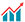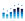A SIMPLE CALCULATION OF GRAVITATIONAL LENSING ON THE ROTATING STARS

Authors

• Norma Sidik Risdianto
• Agung Laksana
• Asih Melati

Keywords:

gravitational lensing, rotating stars, Lagrangian, Kerr metric, Deflection angle

Abstract

We propose a simple calculation to obtain the gravitational lensing on rotating stars. The calculation has been made by using a Lagrangian of Kerr metric and getting  for deflection angle. The calculation has been reduced to slow-rotating stars and equatorials case ()  for maximum deflection angle.Abstract Viewed = 87 times |PDF downloaded = 131 times